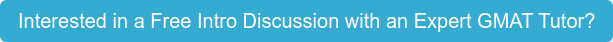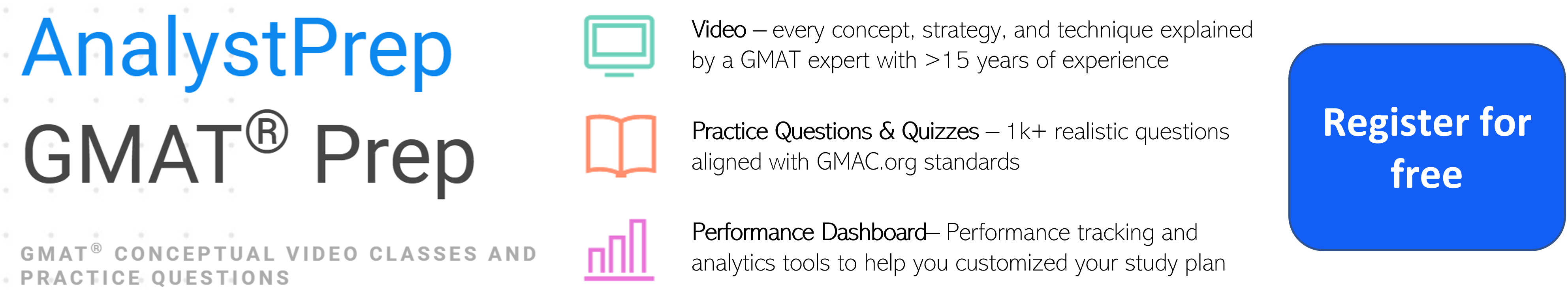# GMAT & MBA Admissions Blog

Mental math is an important skill to have when taking the GMAT (Graduate Management Admission Test). The exam includes a quantitative section that tests math skills, and mental math can help test-takers save valuable time and avoid mistakes.

The GMAT's quantitative section includes both problem-solving questions and data sufficiency questions. In both types of questions, test-takers need to be able to quickly and accurately perform basic arithmetic, such as addition, subtraction, multiplication, and division. Mental math skills can help test-takers avoid errors that might result from using a calculator, and can help them work through problems more quickly.

In addition to the quantitative section, the GMAT also includes an integrated reasoning section that requires the ability to work with data sets and make quick calculations. This section includes questions that require mental math skills, such as determining percentages, ratios, and averages.However, it's important to note that mental math is not the only important skill needed to succeed in the GMAT's quantitative section. Test-takers also need to have a strong foundation in math concepts and problem-solving strategies. It's important to review algebra, geometry, and other math concepts to ensure a solid understanding of the material.

While mental math can be helpful on the GMAT, it's important to note that the exam does allow the use of a calculator. However, the calculator is a simple four-function calculator and does not have the advanced functions of a graphing calculator. Additionally, using a calculator can be time-consuming, so it's important to know when mental math is a more efficient approach.

In summary, mental math is an important skill for success on the GMAT. It can help test-takers save time and avoid errors, and is particularly useful in the quantitative and integrated reasoning sections of the exam. However, mental math is not the only important skill needed to succeed on the GMAT, and test-takers also need a strong foundation in math concepts and problem-solving strategies. One of the best ways to master the problem-solving strategies necessary to excel on the GMAT is to hire a GMAT tutor